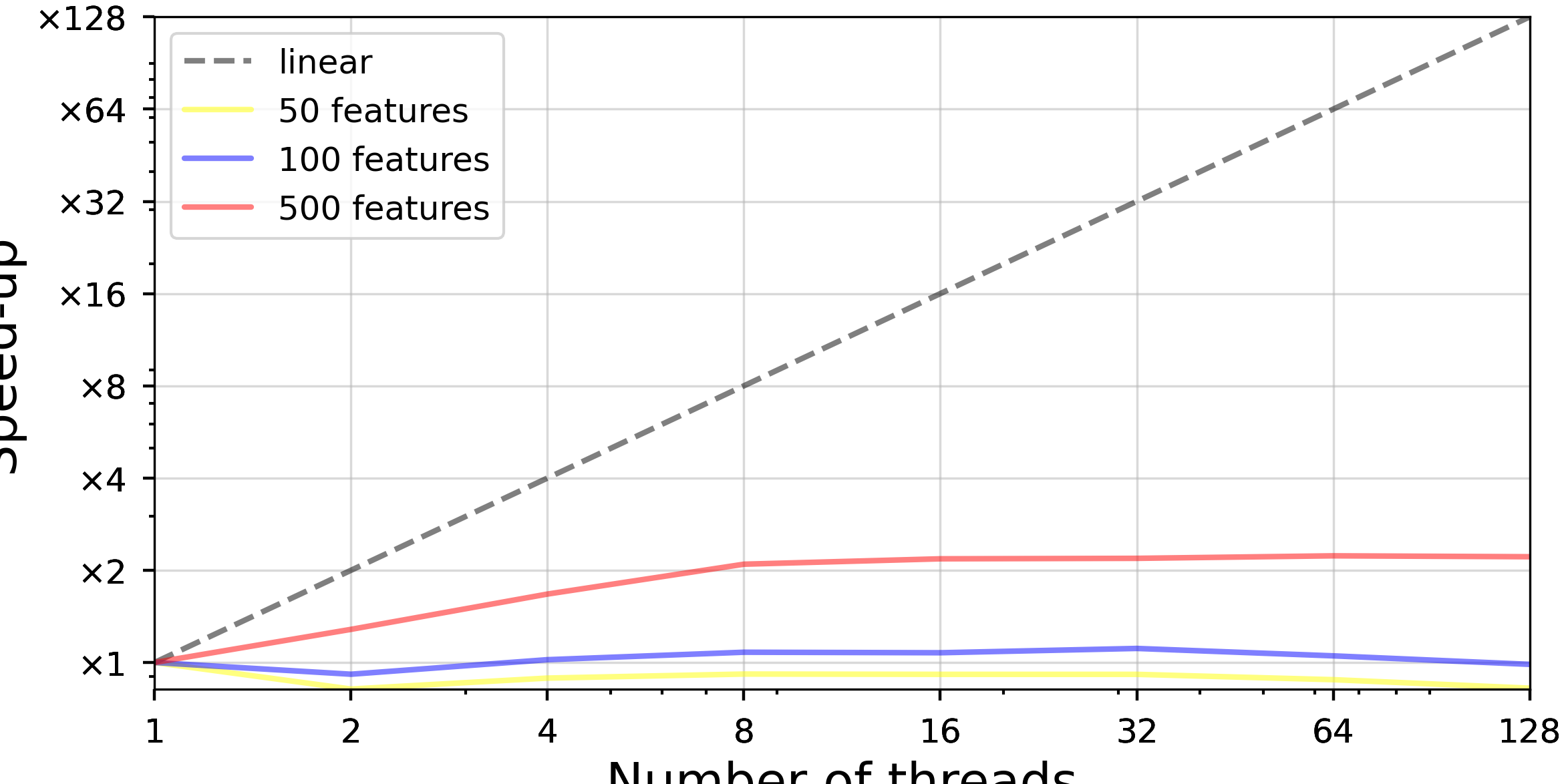Performances and scikit-learn: k-nearest neighbors search

An example of room for improvements

Published on the: 13.01.2022
Estimated reading time: ~ 3 min.

$$k$$-nearest neighbors search in scikit-learn

$$k$$-nearest neighbors search is at the base of many implementations used within scikit-learn.

For instance, it is used in Affinity Propagation, BIRCH, Mean Shift, OPTICS, Spectral Clustering, TSNE, KNeighbors Regressor and KNeighbors Classifier.

Whilst many libraries implement approximated versions of $$k$$-nearest neighbors search to speed-up the time of execution1,

scikit-learn’s implementation aims at returning the exact $$k$$-nearest neighbors.

There are two main aspects in scikit-learn current implementation of $$k$$-nearest neighbors search.

Computing chunks of the distance matrix computations

The pattern for $$k$$-nearest neighbors computing the tasks is the following one:

• Compute $$\mathbf{D}_d(\mathbf{X}, \mathbf{Y})$$, the distance matrix between the vectors of two arrays $$\mathbf{X}$$ and $$\mathbf{Y}$$
• Reduce rows of $$\mathbf{D}_d(\mathbf{X}, \mathbf{Y})$$ appropriately for the given algorithm: for instance, and as covered in a following subsection, the adapted reductions for $$k$$-nn search is $$\text{argkmin}$$ which returns the $$k$$ smallest indices of values in a ordered set.
• Perform extra operations on results of the reductions (here sort values)

Generally, one does not compute $$\mathbf{D}_d(\mathbf{X}, \mathbf{Y})$$ entirely because its space complexity is $$\Theta(n_x \times n_y)$$: technically, $$\mathbf{D}_d(\mathbf{X}, \mathbf{Y})$$ does not fit in RAM for sound datasets.

In practice one thus computes chunks of this dataset which are themselves reduced in streaming. This is what is currently done in scikit-learn2.

Reducing distances: argkmin

The $$\text{argkmin}$$ consist in returning the indices of the $$k$$ smallest value of an ordered container.

In the case of the $$k$$-nearest neighbors search, we can apply $$\text{argkmin}$$ on each row of the distances matrix to get the

Current issues

Unfortunately, most of those reductions are implementated using python functions as reductions functions in a general parallelisation pattern which relies on joblib.Parallel.

This technically is not the most efficient: working at this level with views on numpy arrays moves large chunks of data back and forth several time between the RAM and the CPUs’ caches, hardly make use of caches, and allocate temporary results.

Moreover, the cost of manipulating those chunks of data the CPython interpreter causes a non negligeable overhead because they are associated to Python objects which are bound to the Global Lock Interpreter for their reference counting.

Hence, this does not allow getting proper hardware scalability:1. Approximate nearest neighbors search algorithms comes in many different flavours, and there’s even a benchmark suite comparing them!.
2. KNearestNeighbors.kneighbors is the interface to look for.